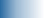### Communication

Mail:
Department of mathematics
Faculty of mathematics and physics
University of Ljubljana
Room:
J21-4.05
Email:
janez.mrcun@fmf.uni-lj.si
Telephone:
+386 1 4766688

### ResearchInterests:
foliations, Lie groupoids and Lie algebroids, differential geometry and topology, algebraic topology
Publications:
• Convolution bialgebra of a Lie groupoid and transversal distributions (with J. Kalisnik)
J. Geom. Phys. 180 (2022), 104642. (MathSciNet) (zbMATH)
• Monodromy and faithful representability of Lie groupoids.
Topology Appl. 240 (2018), 137–158. (MathSciNet) (zbMATH)
• Homotopy sequence of a topological groupoid with a basegroup and an obstruction to presentability of proper regular Lie groupoids. (with B. Jelenc)
J. Homotopy Relat. Struct. 10 (2015), 519–536. (MathSciNet) (zbMATH)
• A Cartier-Gabriel-Kostant structure theorem for Hopf algebroids. (with J. Kalisnik)
Adv. Math. 232 (2013), 295–310. (MathSciNet) (zbMATH)
• On the universal enveloping algebra of a Lie algebroid. (with I. Moerdijk)
Proc. Amer. Math. Soc. 138 (2010), 3135–3145. (MathSciNet) (zbMATH)
• Equivalence between the Morita categories of etale Lie groupoids and of locally grouplike Hopf algebroids. (with J. Kalisnik)
Indag. Math. 19 (2008), 73–96. (MathSciNet) (zbMATH)
• Sheaf coalgebras and duality.
Topology Appl. 154 (2007), 2795–2812. (MathSciNet) (zbMATH)
• Multiplicative bijections between algebras of differentiable functions. (with P. Semrl)
Ann. Acad. Sci. Fenn. Math. 32 (2007), 471–480. (MathSciNet) (zbMATH)
• On duality between etale groupoids and Hopf algebroids.
J. Pure Appl. Algebra 210 (2007), 267–282. (MathSciNet) (zbMATH)
• On the developability of Lie subalgebroids. (with I. Moerdijk)
Adv. Math. 210 (2007), 1–21. (MathSciNet) (zbMATH)
• On the integrability of Lie subalgebroids. (with I. Moerdijk)
Adv. Math. 204 (2006), 101–115. (MathSciNet) (zbMATH)
• On isomorphisms of algebras of smooth functions.
Proc. Amer. Math. Soc. 133 (2005), 3109–3113. (MathSciNet) (zbMATH)
• Lie groupoids, sheaves and cohomology. (with I. Moerdijk)
Poisson Geometry, Deformation Quantisation and Group Representations, 145–272, London Math. Soc. Lecture Note Ser. 323, Cambridge University Press, Cambridge, 2005. (abstract) (MathSciNet) (zbMATH)
• Introduction to Foliations and Lie Groupoids. (with I. Moerdijk)
Cambridge Studies in Advanced Mathematics 91, Cambridge University Press, Cambridge, 2003. (abstract) (errata) (MathSciNet) (zbMATH)
• On integrability of infinitesimal actions. (with I. Moerdijk)
Amer. J. Math. 124 (2002), 567–593. (MathSciNet) (zbMATH)
• The Hopf algebroids of functions on etale groupoids and their principal Morita equivalence.
J. Pure Appl. Algebra 160 (2001), 249–262, erratum ibid. 176 (2002), 275-276. (MathSciNet) (zbMATH)
• Functoriality of the bimodule associated to a Hilsum-Skandalis map.
K-Theory 18 (1999), 235–253. (MathSciNet) (zbMATH)
• On the stability of equivariant foliations.
Proc. Amer. Math. Soc. 125 (1997), 1561–1570. (MathSciNet) (zbMATH)
• An extension of the Reeb stability theorem.
Topology Appl. 70 (1996), 25–55. (MathSciNet) (zbMATH)
• Lipschitz spectrum preserving mappings on algebras of matrices.
Linear Algebra Appl. 215 (1995), 113–120. (MathSciNet) (zbMATH)Updated: October 22, 2022